KMP算法 内部涉及到的数学原理与知识太多，本文只会对 KMP算法 的运行过程、 部分匹配表next数组 进行介绍，如果理解了这三点再去阅读其它有关 KMP算法 的文章肯定能有个清晰的认识。

### 定义

Knuth-Morris-Pratt 字符串查找算法，简称为 KMP算法，常用于在一个文本串 S 内查找一个模式串 P 的出现位置。

KMP算法 的操作流程如下：

• 假设现在文本串 S 匹配到 i 位置，模式串 P 匹配到 j 位置
• 如果 j = -1，或者当前字符匹配成功（即 S[i] == P[j] ），都令 i++，j++，继续匹配下一个字符；
如果 j != -1，且当前字符匹配失败（即 S[i] != P[j] ），则令 i 不变，j = next[j]。此举意味着失配时，模式串 P相对于文本串 S 向右移动了 j – next [j]  位
• 换言之，将模式串 P 失配位置的 next 数组的值对应的模式串 P 的索引位置移动到失配处

### 运行过程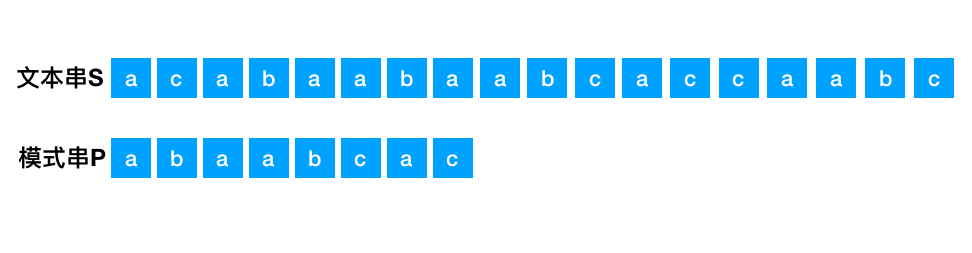a
a b
a b a
a b a a
a b a a b
a b a a b c
a b a a b c a
a b a a b c a c

a
a b
a b a
a b a a

b
a b
a a b
b a a b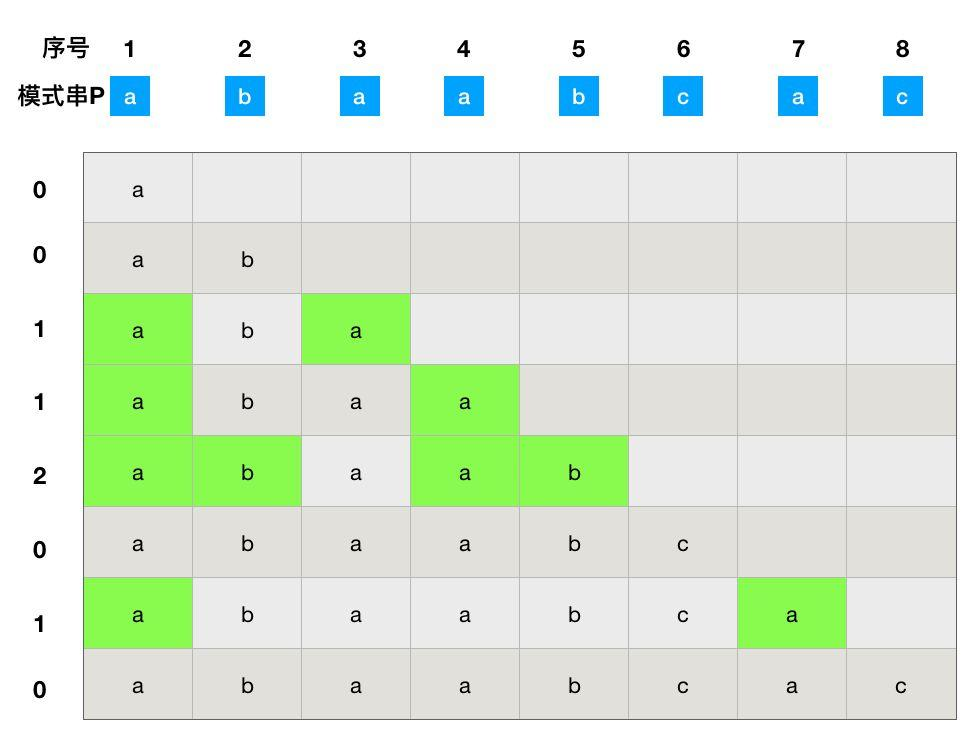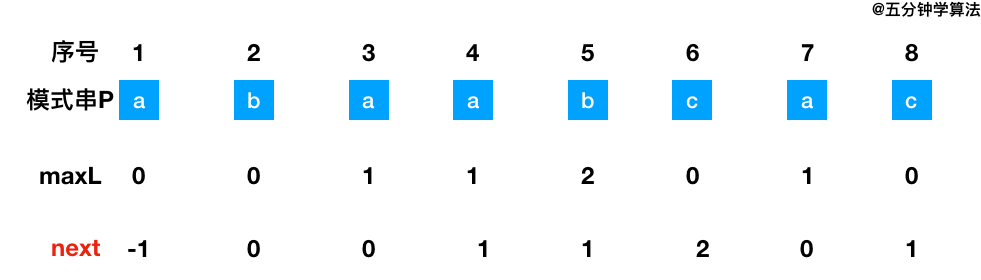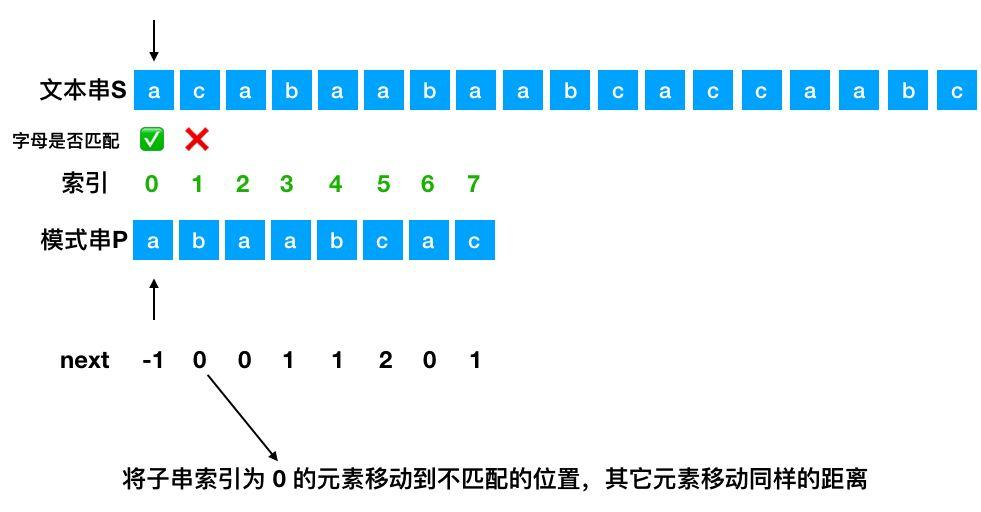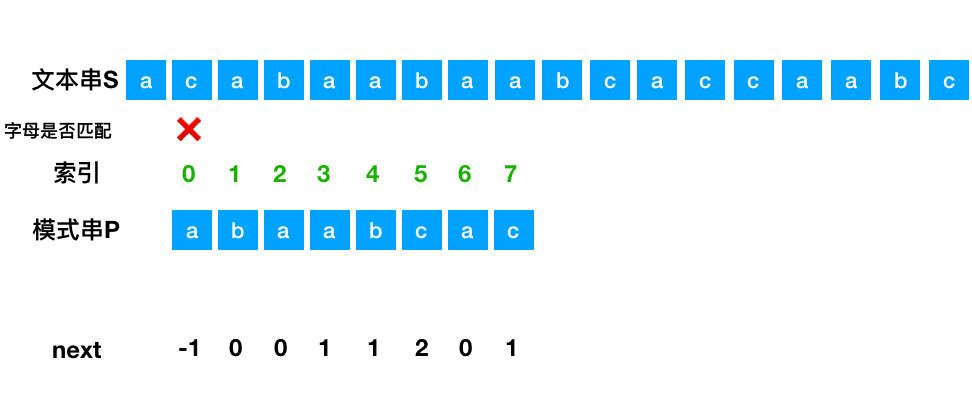### 后记GitHub 标星 3w+，很全面的算法和数据结构知识